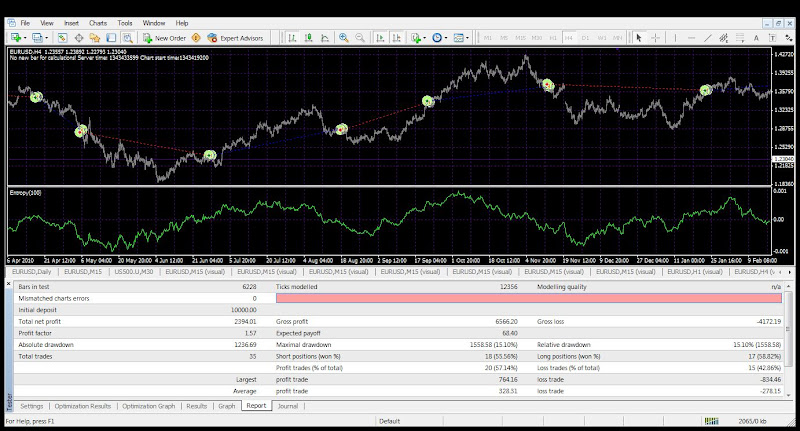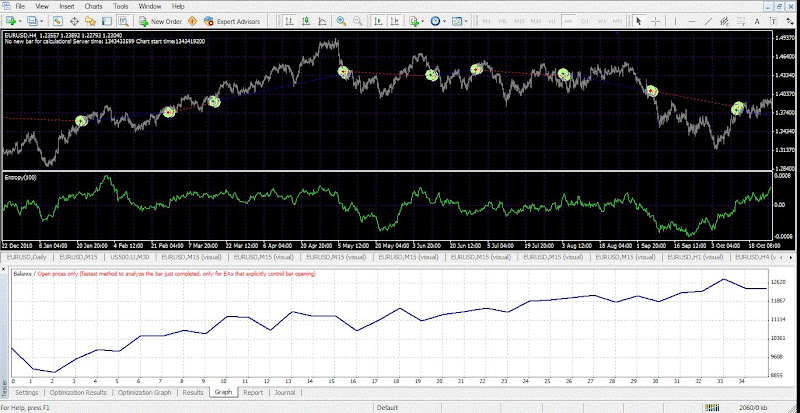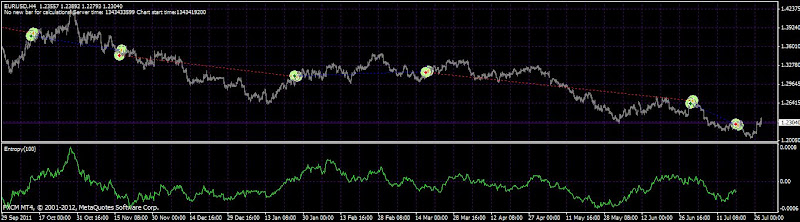## Combining Entropymath with a PNN Expert adviser (Probabilistic Neural Network)

By JohnLast 2813 days ago

What about a trend following system with high number of profitable trades! Is that possible?

No if we use the common waves to do so moving averages and common indicators.

Maybe, if we use a different approach?!

Tonight I was working on the idea of combining the Entropymath concept with the PNN concept.

The Entropymath concept comes from the work of John Conover. The EntropyMath indicator is coded for metatrader 4 and it calculated the g

g means: the average multiplicative gain per iteration of the game, i.e., after `t` many iterations, the gambler's capital would have increased by a factor of `g^t`.

If the parameter numbars is low as it is the default options the indicator works as any other oscillator.

However if we apply a bigger numbers for the calculations then we would have more meaningfull results.

However what strikes me is that in the article the author is talking about If n has a sufficiently large data set size, then by the law of large numbers, in `n` many iterations of the game.

In the excel program they are useing 60 bars as default parameter.

Then the second idea was, why not to combine this model with the PNN model.

And yes it was just one piece of code. I was pleased to see upward equity curve on the 1h and 4 h time frame just by putting the parameters coming from my idea. No optimization.От EA testsОт EA testsОт EA tests